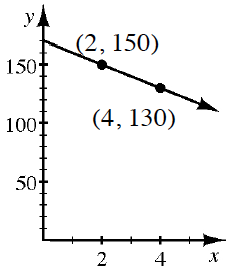### Home > CCA2 > Chapter A > Lesson A.2.1 > ProblemA-55

A-55.

​Draw a slope triangle and use it to find the equation of the line shown in the graph at right.

Find the slope and $y$-intercept.

$y = -10x + 170$